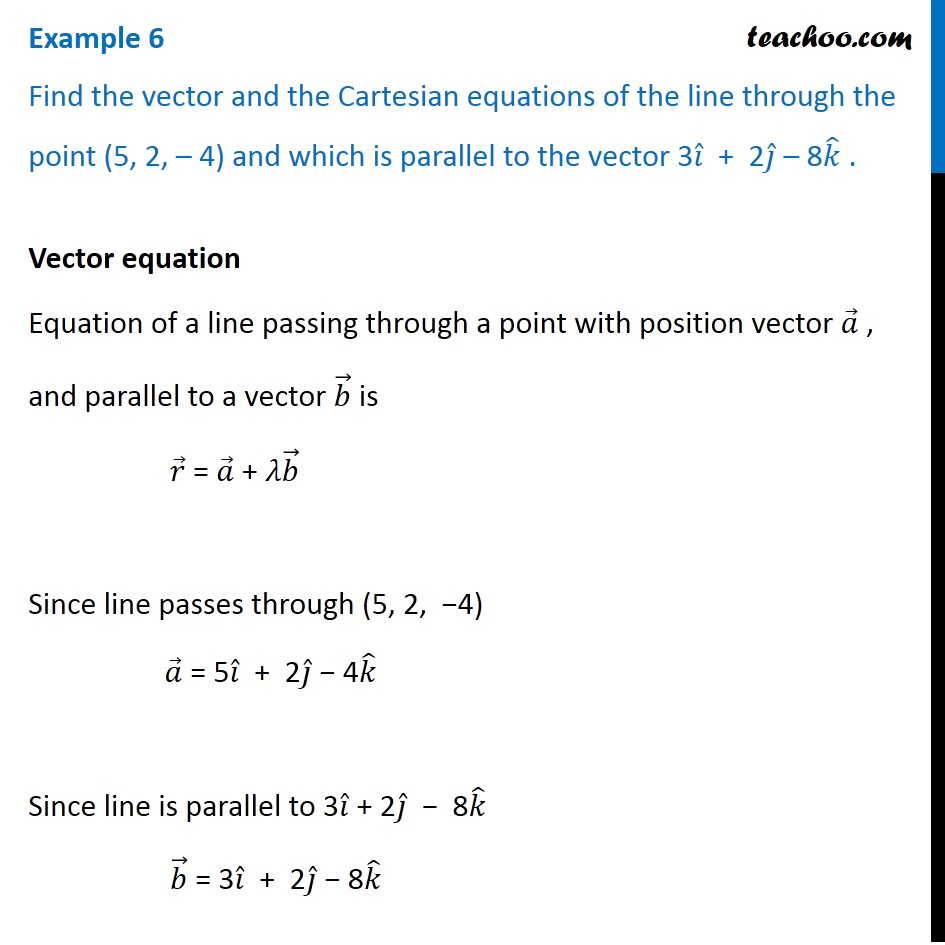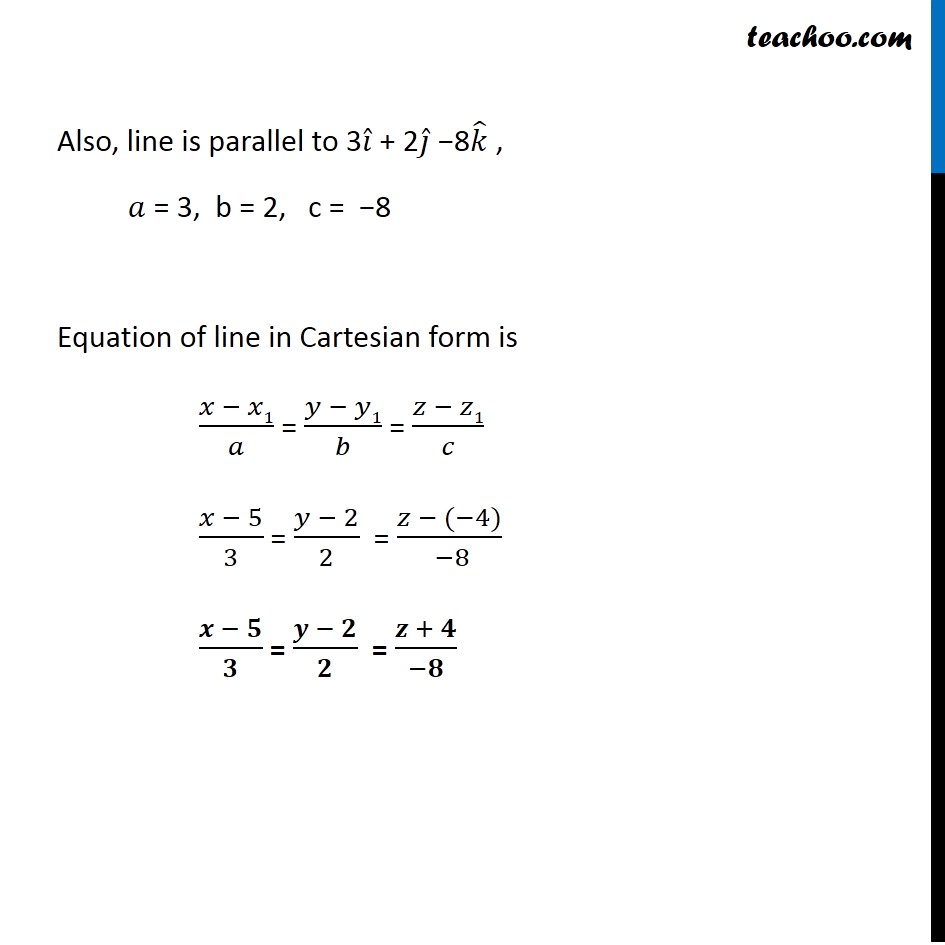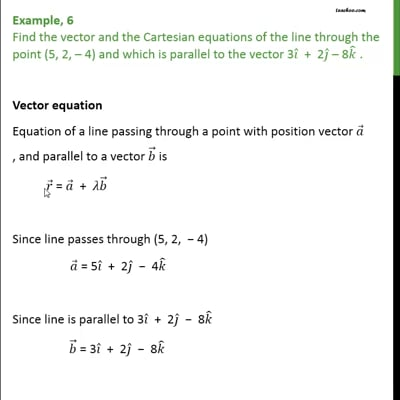Examples

Chapter 11 Class 12 Three Dimensional Geometry
Serial order wiseThis video is only available for Teachoo black users

Solve all your doubts with Teachoo Black (new monthly pack available now!)

### Transcript

Example 6 Find the vector and the Cartesian equations of the line through the point (5, 2, – 4) and which is parallel to the vector 3𝑖 ̂ + 2𝑗 ̂ – 8𝑘 ̂ . Vector equation Equation of a line passing through a point with position vector 𝑎 ⃗ , and parallel to a vector 𝑏 ⃗ is 𝑟 ⃗ = 𝑎 ⃗ + 𝜆𝑏 ⃗ Since line passes through (5, 2, −4) 𝑎 ⃗ = 5𝑖 ̂ + 2𝑗 ̂ − 4𝑘 ̂ Since line is parallel to 3𝑖 ̂ + 2𝑗 ̂ − 8𝑘 ̂ 𝑏 ⃗ = 3𝑖 ̂ + 2𝑗 ̂ − 8𝑘 ̂ Equation of line 𝑟 ⃗ = 𝑎 ⃗ + 𝜆𝑏 ⃗ 𝒓 ⃗ = (5𝒊 ̂ + 2𝒋 ̂ − 4𝒌 ̂) + 𝜆 (3𝒊 ̂ + 2𝒋 ̂ − 8𝒌 ̂) Therefore, equation of line in vector form is 𝑟 ⃗ = (5𝑖 ̂ + 2𝑗 ̂ − 4𝑘 ̂) + 𝜆 (3𝑖 ̂ + 2𝑗 ̂ − 8𝑘 ̂) Cartesian equation Equation of a line passing through a point (x, y, z) and parallel to a line with direction ratios a, b, c is (𝑥 − 𝑥1)/𝑎 = (𝑦 − 𝑦1)/𝑏 = (𝑧 − 𝑧1)/𝑐 Since line passes through (5, 2, −4) 𝑥1 = 5, y1 = 2 , z1 = −4 .....................................